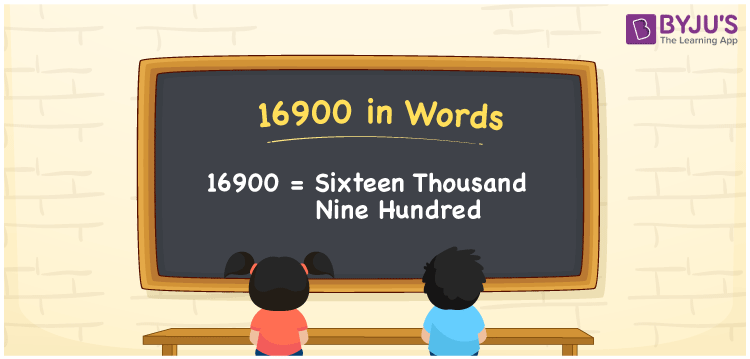# 16900 in Words

16900 in words is written as Sixteen thousand nine hundred. In both the International System of Numerals and the Indian System of Numerals, 16900 is written as Sixteen thousand nine hundred. The number 16900 is a Cardinal Number as it represents some quantity. For example, “the Smart-TV costs 16900 rupees”, 16900 represents the cost of the TV.

 16900 in Words Sixteen thousand nine hundred Sixteen thousand nine hundred in Number 16900

## 16900 in English Words

16900 in English words is read as “Sixteen thousand nine hundred.”## How to Write 16900 in Words?

To write 16900 in words, we shall use the place value chart. In the place value chart, put 1 in the ten thousands, 6 in the thousands, 9 in hundreds, and 0 in the tens and the ones, respectively. Let us make a place value chart to write the number 16900 in words.

 Ten Thousands Thousands Hundreds Tens Ones 1 6 9 0 0

Thus, we can write the expanded form as

1 × Ten Thousand + 6 × Thousand + 9 × Hundred + 0 × Ten + 0 × One

= 1 × 10000 + 6 × 1000 + 9 × 100 + 0 × 10 + 0 × 1

= 10000 + 6000 + 900 + 0 + 0

= 16900

= Sixteen thousand nine hundred.

16900 is a natural number, the successor of 16899 and the predecessor of 16901.

16900 in words – Sixteen thousand nine hundred

• Is 16900 an odd number? – No
• Is 16900 an even number? – Yes
• Is 16900 a perfect square number? – Yes, 130 × 130 = 16900
• Is 16900 a perfect cube number? – No
• Is 16900 a prime number? – No
• Is 16900 a composite number? – Yes

## Frequently Asked Questions on 16900 in Words

Q1

### How to write 16900 in words?

16900 in words is written as Sixteen thousand nine hundred.
Q2

### How to write 16900 in the International and Indian System of Numerals?

In both, the system of numerals, 16900 in words, is written as Sixteen thousand nine hundred.
Q3

### How to write 16900 in a place value chart?

In the place value chart, write 1 in the ten thousands, 6 in the thousands, 9 in hundreds, and 0 in the tens and the ones, respectively.